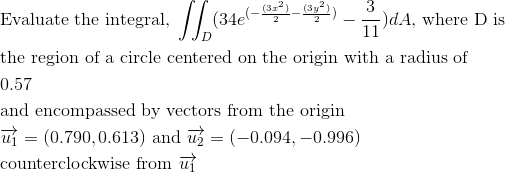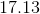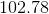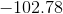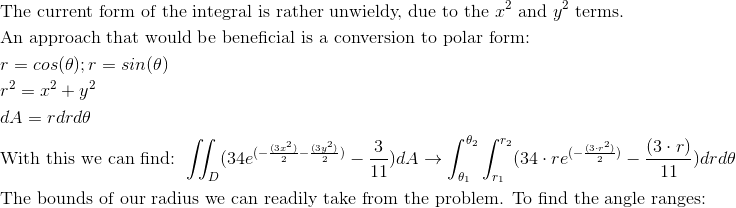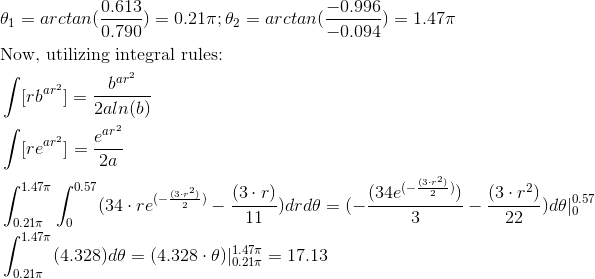Calculus 3 : Double Integrals

Example Questions

Example Question #31 : Double Integration In Polar Coordinates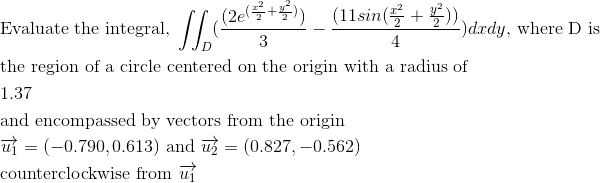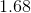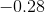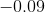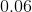Explanation: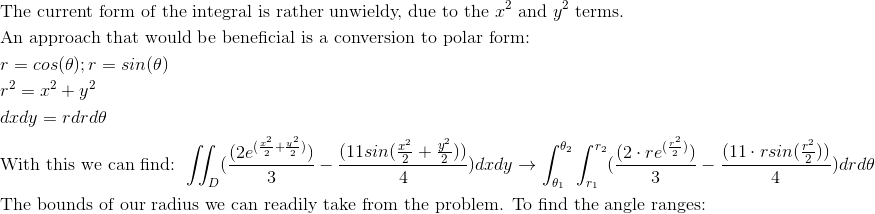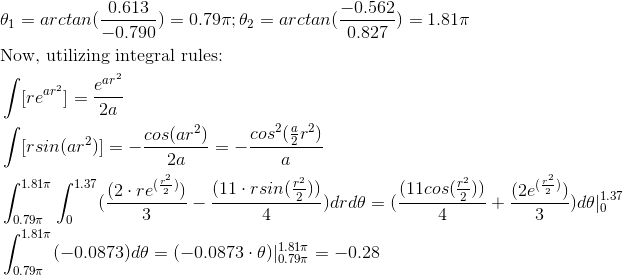Example Question #32 : Double Integration In Polar Coordinates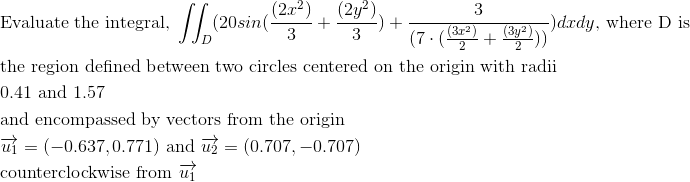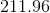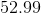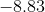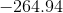Explanation: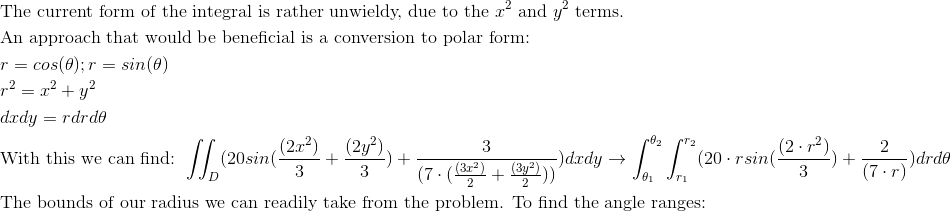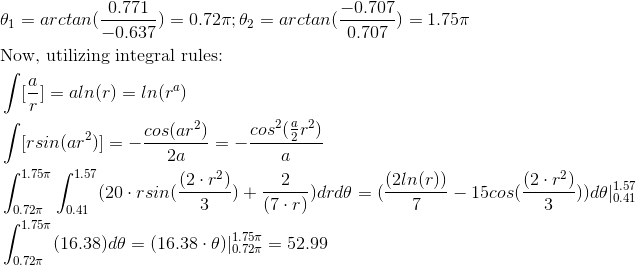Example Question #33 : Double Integration In Polar Coordinates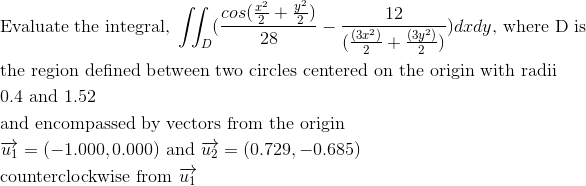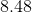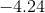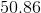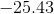Explanation: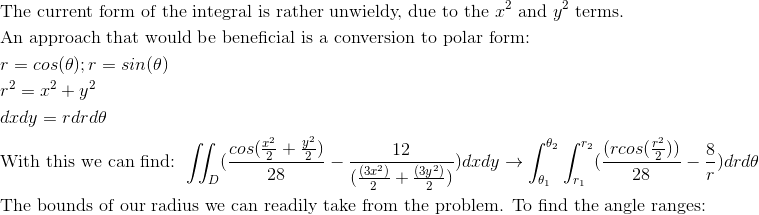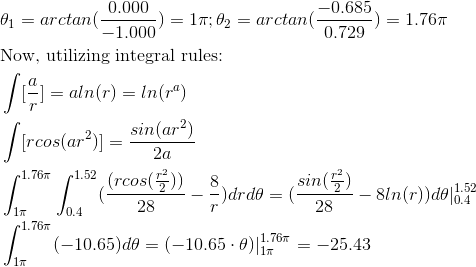Example Question #34 : Double Integration In Polar Coordinates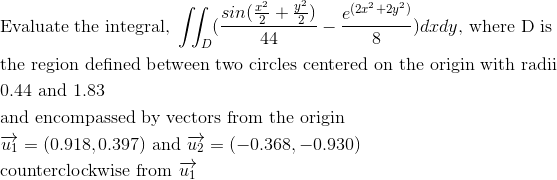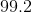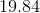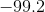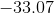Explanation: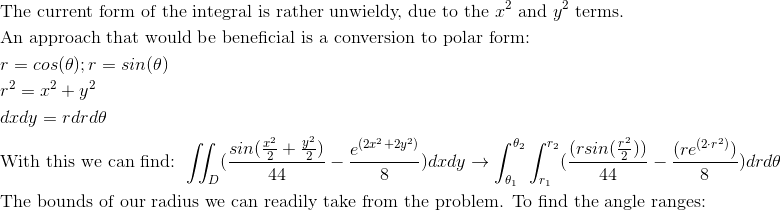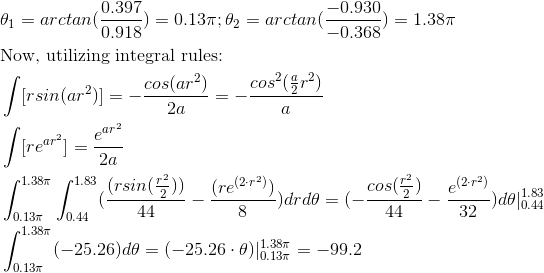Example Question #35 : Double Integration In Polar Coordinates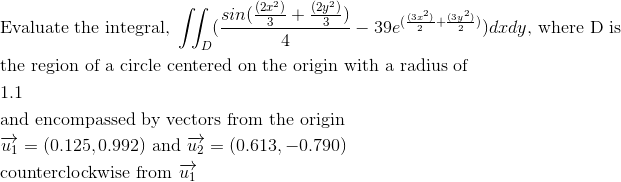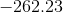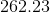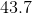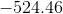Explanation: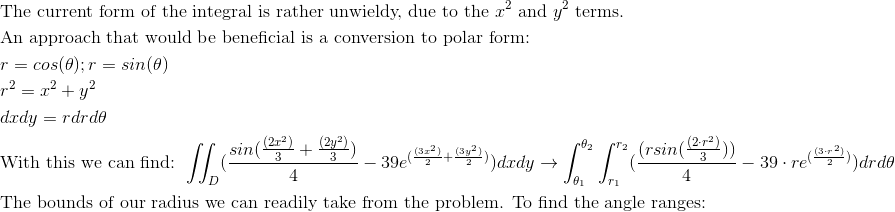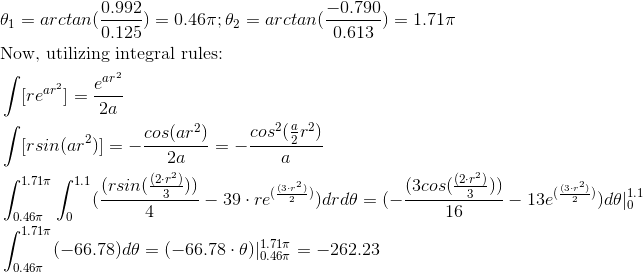Example Question #36 : Double Integration In Polar Coordinates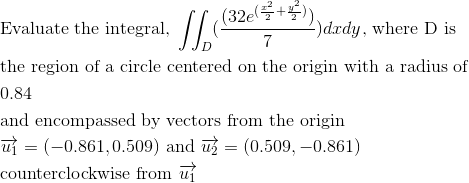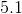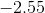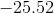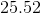Explanation: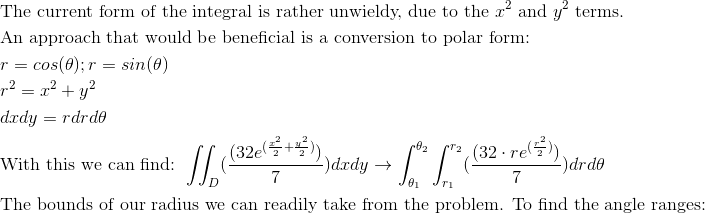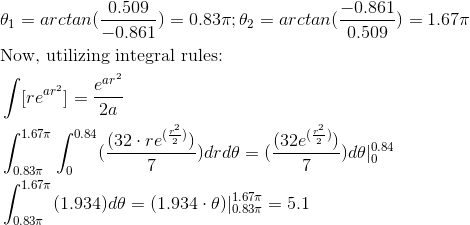Example Question #37 : Double Integration In Polar Coordinates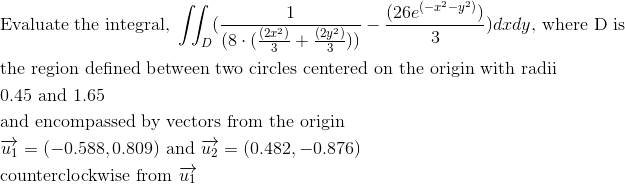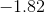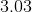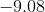Explanation: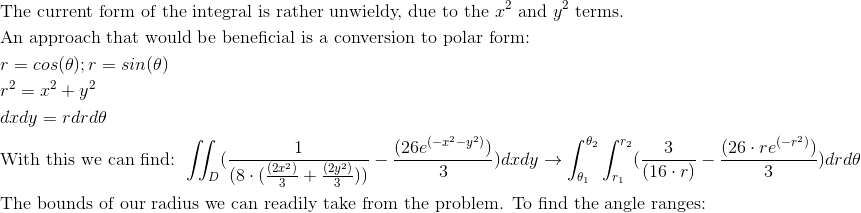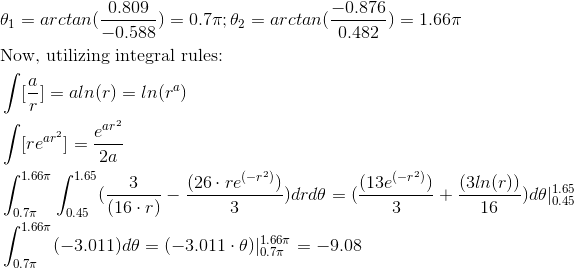Example Question #38 : Double Integration In Polar Coordinates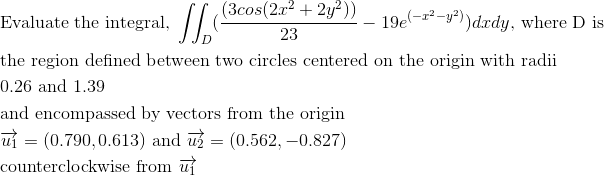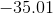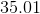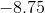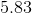Explanation: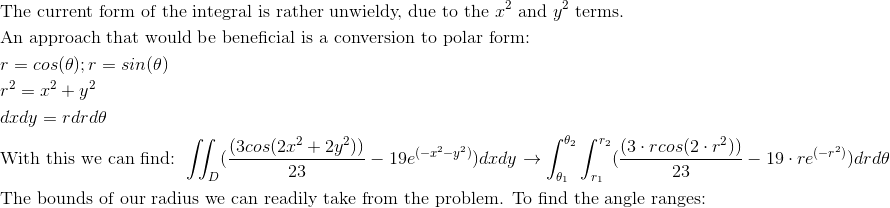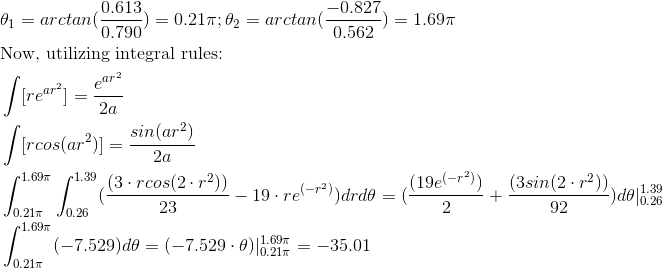Example Question #39 : Double Integration In Polar Coordinates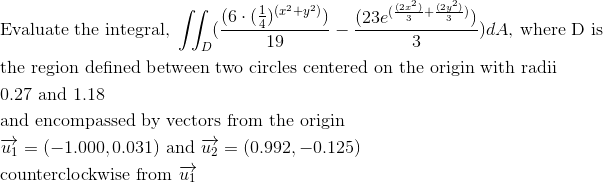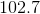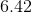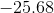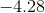Explanation: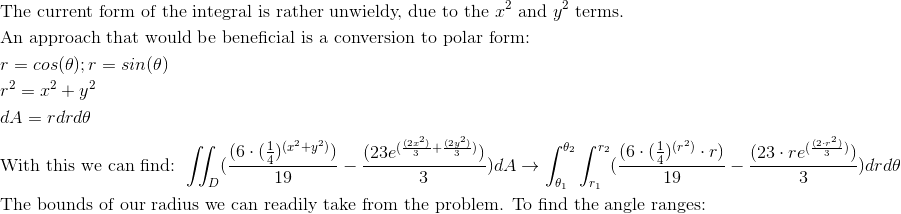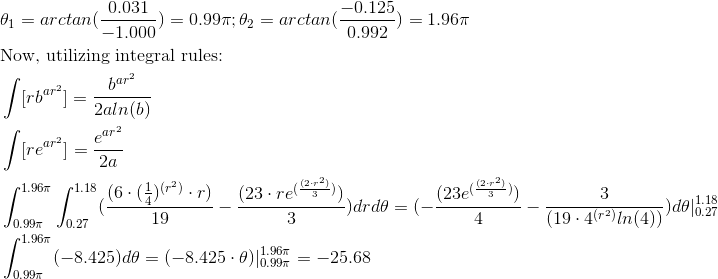Example Question #40 : Double Integration In Polar Coordinates